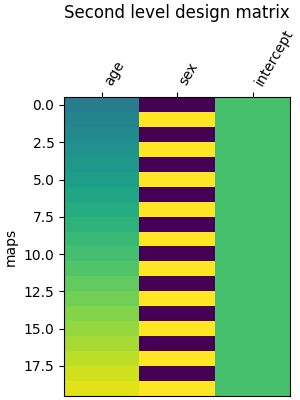# Example of second level design matrix#

This example shows how a second-level design matrix is specified: assuming that the data refer to a group of individuals, with one image per subject, the design matrix typically holds the characteristics of each individual.

This is used in a second-level analysis to assess the impact of these characteristics on brain signals.

This example requires matplotlib.

```try:
import matplotlib.pyplot as plt
except ImportError:
raise RuntimeError("This script needs the matplotlib library")
```

## Create a simple experimental paradigm#

We want to get the group result of a contrast for 20 subjects.

```n_subjects = 20
subjects_label = [f"sub-{int(i):02}" for i in range(1, n_subjects + 1)]
```

Next, we specify extra information about the subjects to create confounders. Without confounders the design matrix would correspond to a one sample test.

```import pandas as pd

extra_info_subjects = pd.DataFrame(
{
"subject_label": subjects_label,
"age": range(15, 15 + n_subjects),
"sex": [0, 1] * (n_subjects // 2),
}
)
```

## Create a second level design matrix#

With that information we can create the second level design matrix.

```from nilearn.glm.second_level import make_second_level_design_matrix

design_matrix = make_second_level_design_matrix(
subjects_label, extra_info_subjects
)
```
```/home/yasmin/nilearn/nilearn/nilearn/glm/first_level/design_matrix.py:499: UserWarning:

Attention: Design matrix is singular. Aberrant estimates are expected.
```

Let’s plot it.

```from nilearn.plotting import plot_design_matrix

ax = plot_design_matrix(design_matrix)
ax.set_title("Second level design matrix", fontsize=12)
ax.set_ylabel("maps")
plt.tight_layout()
plt.show()
```Total running time of the script: ( 0 minutes 2.930 seconds)

Estimated memory usage: 8 MB

Gallery generated by Sphinx-Gallery